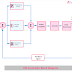## What is PID Controller?

PID controller is a device built with a closed-loop control system that performs proportionally, integral, and derivative controls. The full form of PID Controller is Proportional Integral Derivative Controller. It is an advanced and complex controlling system used in industrial process and control applications. PID controller can work with different process variables such as temperature, speed, pressure, etc. In the modulated control system, the PID controller helps a lot. The PID controlling system has a feedback path and it takes feedback signals from the output to input that is why it can be called as a closed-loop control system.

## PID Controller Block Diagram

Here, you can see the block diagram of the PID Controller. It will help a lot to understand the working principle of PID Controller.

In the above block diagram, each part are shown properly,
• Reference or Setpoint
• Proportional Action
• Integral Action
• Derivative Action
• Power Amplifier
• Actuator
• Plant Operation or Structure
• Actual Response or Output
• Feedback Path
• Summing Points

## How PID Controller Works?

The PID Controller is a Closed Loop control system. It takes the feedback signal from the output to input and by comparing the output with input it generates the Error Signal. Now the error signal is processed and fed to the output. As we know it works on three basic principles.

### Proportional(P)

It works with the principle of proportionality. That means its output is proportional to the actual value of the error signal. If the error signal is large, the control output also be large, and if the error signal is small the control output also be small. Here, the ratio of output to input is known as the gain factor which is also known as the proportional factor.

### Integral(I)

In this section, the controller integrates the actual value of the error signal and tries to decrease the steady-state error. Because of the integration process, the integral part or very small value of the error signal make results in a very high integral response. The controlling integration continuously happens until the error becomes zero.

### Derivative(D)

In this controlling section, the output is directly proportional to the rate of change of error with respect to time. So it clearly shows that it will provide an output when the error is changing. For constant error value, it will cause the output zero. It may so there are no errors but in actuality, there are errors available(constant). So it is always working with the combination of PD or PID, it cannot be performed alone as its output value will be zero.

## PID Controller with Example

Advanced temperature controller, car speed controller, etc are examples of PID Controller.

## PID Controller Types

There are main types of PID Controller are,
1. On/Off Controller
2. Proportional Controller
3. Standard Type PID Controller
4. Real-Time PID Controller

## Use and Applications of PID Controller

1. Temperature Controlling: In advanced temperature controlling systems, PID controller is widely used. Industrial temperature controlling for furnace, HVAC system, etc PID controllers are used.

2. Automatic Machine Control: In the automatic machine control system, such as production counting, cutting, etc purpose also PID controller is used.

3. Automobile Industry: In the automobile industry also PID controllers are widely used such as car speed measurement, automatic driving, etc.

4. Power Electronic Circuits or Devices: In power electronic circuits such as converter, inverter, voltage stability, the PID controller is used.

## Advantages and Disadvantages of PID Controller

1. PID controller provides a very high-efficiency steady-state output than normal on/off controllers.

2. As the PID controller works in three modes, so the error minimizing, data validation is easily possible.

PID Controller Block Diagram, Working Principle, Example, UseReviewed by Author on 2/07/2022 Rating: 5